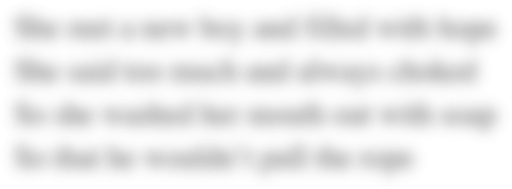# MAT 540 Week 9 Quiz 5

Quiz 5

Question 1

2 out of 2 points

 In a _______ integer model, some solution values for decision variables are integer and others can be non-integer.

Question 2

2 out of 2 points

 In a total integer model, some solution values for decision variables are integer and others can be non-integer.

Question 3

2 out of 2 points

 In a problem involving capital budgeting applications, the 0-1 variables designate the acceptance or rejection of the different projects.

Question 4

2 out of 2 points

 If a maximization linear programming problem consist of all less-than-or-equal-to constraints with all positive coefficients and the objective function consists of all positive objective function coefficients, then rounding down the linear programming optimal solution values of the decision variables will ______ result in a(n)  _____ solution to the integer linear programming problem.

Question 5

2 out of 2 points

 The branch and bound method of solving linear integer programming problems is an enumeration method.

Question 6

2 out of 2 points

 In a mixed integer model, all decision variables have integer solution values.

Question 7

2 out of 2 points

 For a maximization integer linear programming problem, feasible solution is ensured by rounding _______  non-integer solution values if all of the constraints are less-than -or equal- to type.

Question 8

2 out of 2 points

 In a total integer model, all decision variables have integer solution values.

Question 9

2 out of 2 points

 The 3 types of integer programming models are total, 0 - 1, and mixed.

Question 10

2 out of 2 points

 The branch and bound method of solving linear integer programming problems is  ________________.

Question 11

2 out of 2 points

 The linear programming relaxation contains the  _______ and the original constraints of the integer programming problem, but drops all integer restrictions. Answer

Question 12

2 out of 2 points

 The branch and bound method can only be used for maximization integer programming problems.

Question 13

2 out of 2 points

 The solution value (Z)  to the linear programming relaxation of a minimization problem  will always be less than or equal to the optimal solution value (Z) of the integer programming minimization problem

Question 14

2 out of 2 points

Question 15

2 out of 2 points

 Types of integer programming models are _____________.

Question 16

2 out of 2 points

 In a 0 - 1 integer model, the solution values of the decision variables are 0 or 1.

Question 17

2 out of 2 points

 Which of the following is not an integer linear programming problem? Answer

Question 18

2 out of 2 points

 In a mixed integer model, some solution values for decision variables are integer and others can be non-integer.

Question 19

2 out of 2 points

 Rounding small values of decision variables to the nearest integer value causes ______________ problems than rounding large values. Answer

Question 20

2 out of 2 points

 In using rounding of a linear programming model to obtain an integer solution, the solution is Answer
Field of study:
Date Due:
Tuesday, October 23, 2018